### C++ general knowledge questions and answers

 (1) Classification of Constructor. Explain with Syntax. (2) Why use Function Overloading?  Explain with an example. (3) Difference between Abstraction and Encapsulation. (4) Is C++ support multiple inheritance? If not, Why not support? If yes, is there any probability to occur ambiguity? If yes, How can remove ambiguity? (5) Explain Polymorphism with Syntax.

1.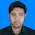Name: Md. Johorul Islam
ID: 201720237
CSE-DIP-55 Batch
-------------------------

2.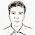Name: Khorshed Alam
ID: 201730438
CSE-DIP-56 Batch
-------------------------

3.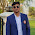Name:Asib Bin Rhaman.
ID:201730160
Dept:CSE(Diploma)
Batch:56th
Campus:Uttara.
----------------------
# CONSTRUCTOR:
------------
A class constructor is a special member function
of a class that is executed whenever we create
new objects of that class.

A constructor will have exact same name as the
class andit does not have any return type at
all, not even void.Constructors can be very useful
for setting initial values for certain member
variables.

constructor can place inside class with parameter,
without parameter ot both of them.

Syntex:
-------
class sum
{
public:
sum(int x,int y)
{
cout<
using namespace std;
class calculator
{
public:
double result = 0;
int match = 0;
void sum(int num1, int num2)
{
result = num1+num2;
output();
}
void sum(int num1, int num2, int num3)
{
result = num1+num2+num3;
output();
}
void sum(double num1, double num2)
{
result = num1+num2;
output();
}
void output()
{
match = result;
if(match == result)
{
cout<<"The result of sum ="<
using namespace std;
class Shape {
protected:
int width, height;
public:
Shape( int a = 0, int b = 0){
width = a;
height = b;
}
int area() {
cout << "Parent class area :" <
using namespace std;
class Shape {
protected:
int width, height;
public:
Shape( int x, int y){
width = x;
height = y;
}
int area() {
cout << "Area of this shape :"<< width*height <area();
shape = &tri;
shape
-
>area();
}
output:
Area of this shape :70
Area of this shape :50

4.CSE-55th Batch
Roll: 201720297

https://pastebin.com/gvAjW2ui

5.Name:Al Hasan
ID: 201730011
CSE-DIP-56 Batch
-------------------------

6.https://www.dropbox.com/home/Programming%20Assignment

7.Fazle Rabbi Khan Taron
201720293
55th

8.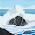9.10.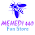mehedi hasan
ID:201730632
56th batch
https://www.dropbox.com/home?preview=assignment.docx

11.Minhazul Abid Sakin
roll: 201720265
batch: 55th

12.Name: Sayeka Jannat
ID: 201720097
CSE- DIP- 55 Batch

1. Classification of Constructor. Explain with Syntax.

Classification of Constructor:
1. Default,
2. Parameterized and
3. Copy Constructors.

Example:
#include
using namespace std;
class construct
{
public:
int a, b;
// Default Constructor
construct()
{
a = 10;
b = 20;
}
};
int main()
{
// Default constructor called automatically
// when the object is created
construct c;
cout << "a: "<< c.a << endl << "b: "<< c.b;
return 1;
}

Function overloading can be considered as an example of polymorphism feature in C++.

Example:
#include
using namespace std;
{
public:
void func(int a,int b)
{
cout<<"sum:"<> a;
cin>>b;
cin>>c;
p.func(a,b);
p.func(a,b,c);
return 0;
}:

3. Difference between Abstraction and Encapsulation.

4. Is C++ support multiple inheritance? If not, Why not support? If yes, is there any probability to occur ambiguity? If yes, How can remove ambiguity?

5. Explain Polymorphism with Syntax.

Polymorphism: The word polymorphism means having many forms. In simple words, polymorphism occurs when there is a hierarchy of classes and they are related by inheritance and a pointer variable in main section. We can define polymorphism as the ability of a message to be displayed in more than one form.

Example:
// pointers to base class
#include
using namespace std;

class Polygon {
protected:
int width, height;
public:
void set_values (int a, int b)
{ width=a; height=b; }
};

class Rectangle: public Polygon {
public:
int area()
{ return width*height; }
};

class Triangle: public Polygon {
public:
int area()
{ return width*height/2; }
};

int main () {
Rectangle rect;
Triangle trgl;
Polygon * ppoly1 = &rect;
Polygon * ppoly2 = &trgl;
ppoly1->set_values (4,5);
ppoly2->set_values (4,5);
cout << rect.area() << '\n';
cout << trgl.area() << '\n';
return 0;
}

Comment Here